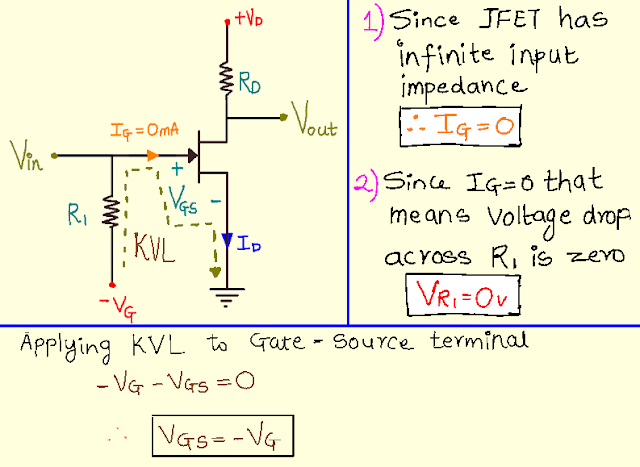### Biasing techniques of JFET

In the last post, we saw the different operating region of JFET, in this post, we are going to learn different biasing techniques of JFET. I hope you read the earlier post. So let's start with this post
but before starting this post I hope that everyone has studied BJT  at least once if not then don't worry but before reading this post please visit my post on what is biasing and the need for biasing transistors? because for biasing you need to understand the concept of load-line and q-point and I had explained this concept in-depth in that post so please do visit that post if you are a beginner or not learned BJT but starting with JFET. Now in JFET biasing is required because of some reasons and that reasons I will be discussing in my next post because there are some parameters and some biasing techniques which we will be required to understand why biasing of JFET are required?
In this particular post, you will be learning three different biasing techniques and at the end, I had inserted one video which explains the working of JFET as an amplifier, and at the same time, it will show you that how the output of JFET is 180 degree out of phase with respect to the input.
So in total, there are 3 biasing techniques of JFET and they are as follows
1) Fixed biasing technique
2) Self-biasing technique and
3) Voltage-divider biasing
You will get to know the working of each biasing technique in great depth but before that please subscribe to my site by clicking on that bell icon. So let's start with the working of each biasing technique of JFET.

1) Fixed biasing technique of JFET:-

As we saw in the previous post that the gate voltage is kept negative so in this technique we are keeping the gate voltage at the fixed point. As we had seen in the previous post that the input impedance of the JFET is very high(theoretically input impedance is infinite and practically in MΩ) therefore the current through resistor R1 will be zero I=0mA thus we can say that the voltage drop across the resistor R1 is 0v that is VR1=0v.2) Self-biasing technique of JFET:-

When we add or basically when we connect the source resistance to a fixed bias circuit then the circuit is known as the self-biasing circuit. Now you will be thinking that why this circuit is known as a self-bias circuit to understand it just look at the figure given below. When we apply KVL to the gate loop we get equation (1) as shown in the figure.

As you can see above we got one equation,
Vgs =  -Id*Rs
So now let's say if Id increases suddenly due to increasing external temperature then from the above equation you can see that the Vgs decreases quickly or in other words Vgs becomes more negative. Since Vgs decreases on its own with an increase in output current Id, therefore, this technique is known as a self-biasing technique.

3) Voltage-Divider Biasing technique of JFET:-

Voltage divider biasing is also known as potential-divider biasing. In this technique, an additional resistor is connected between the supply voltage and gate resistor. The additional gate resistor Rl from gate to supply voltage facilitates the larger adjustment of the dc bias point. Also, remember one thing that while designing the amplifier with this circuit the source voltage must be greater than the voltage across R2 so that the Vg will always be negative. Thus by using this technique, we are able to change the Vgs according to our will, and thus by using this single circuit we can actually use it for different applications such as a switch or as an amplifier.

# CONCLUSION:-
So as you saw above in voltage divider bias that the R1 was providing larger adjustment of the dc bias point also R2 was acting like a self-bias circuit due to this reasons Voltage divider bias is preferred more than any biasing technique.
Now,
In all the above images you are seeing that the output of JFET is an amplified form of input but it is 180 degrees out of phase with respect to the input signal.
Why the output of JFET is 180 degree out of phase w.r.t to input?
For better understanding please do watch the video given below.
VIDEO:-